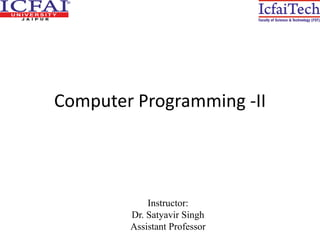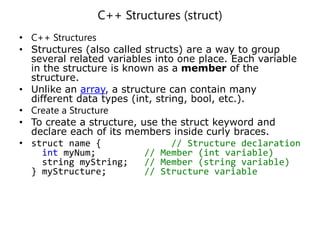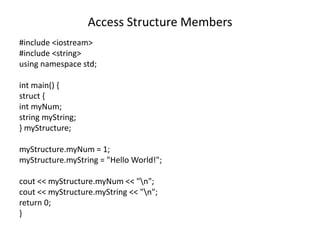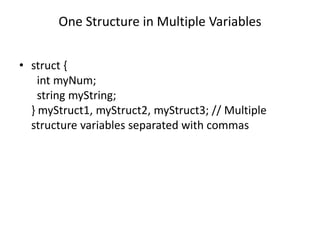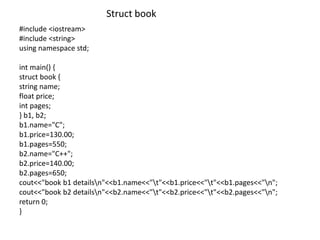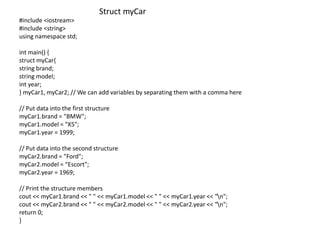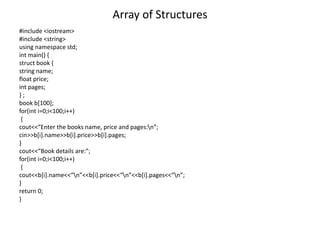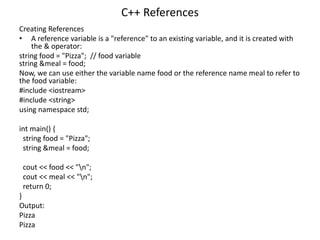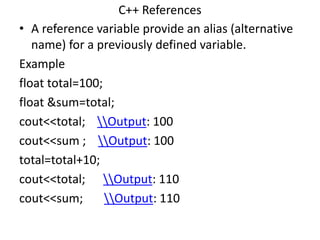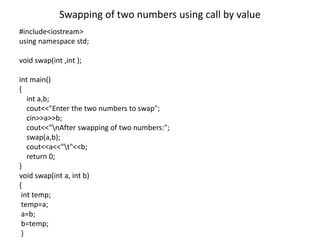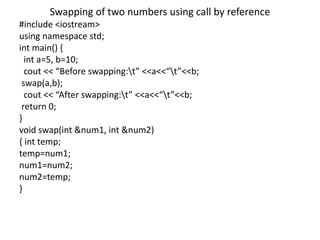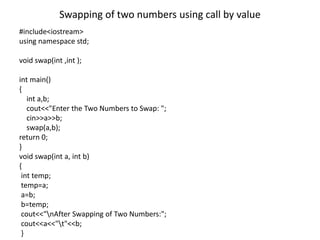1 sur 12

## RecommandéCs1123 12 structuresTAlha MAlik[OOP - Lec 13,14,15] Constructors / Destructor and its TypesMuhammad Hammad WaseemCHAPTER -4-class and structure.pptxGebruGetachew2Structure & Union in C++Davinder KaurCh7 structuresHattori Sidek

### Computer Programming -II (Lec. 10).pptx

1. Computer Programming -II Instructor: Dr. Satyavir Singh Assistant Professor
2. C++ Structures (struct) • C++ Structures • Structures (also called structs) are a way to group several related variables into one place. Each variable in the structure is known as a member of the structure. • Unlike an array, a structure can contain many different data types (int, string, bool, etc.). • Create a Structure • To create a structure, use the struct keyword and declare each of its members inside curly braces. • struct name { // Structure declaration int myNum; // Member (int variable) string myString; // Member (string variable) } myStructure; // Structure variable
3. Access Structure Members #include <iostream> #include <string> using namespace std; int main() { struct { int myNum; string myString; } myStructure; myStructure.myNum = 1; myStructure.myString = "Hello World!"; cout << myStructure.myNum << "n"; cout << myStructure.myString << "n"; return 0; }
4. One Structure in Multiple Variables • struct { int myNum; string myString; } myStruct1, myStruct2, myStruct3; // Multiple structure variables separated with commas
5. #include <iostream> #include <string> using namespace std; int main() { struct book { string name; float price; int pages; } b1, b2; b1.name="C"; b1.price=130.00; b1.pages=550; b2.name="C++"; b2.price=140.00; b2.pages=650; cout<<"book b1 detailsn"<<b1.name<<"t"<<b1.price<<"t"<<b1.pages<<"n"; cout<<"book b2 detailsn"<<b2.name<<"t"<<b2.price<<"t"<<b2.pages<<"n"; return 0; } Struct book
6. #include <iostream> #include <string> using namespace std; int main() { struct myCar{ string brand; string model; int year; } myCar1, myCar2; // We can add variables by separating them with a comma here // Put data into the first structure myCar1.brand = "BMW"; myCar1.model = "X5"; myCar1.year = 1999; // Put data into the second structure myCar2.brand = "Ford"; myCar2.model = “Escort"; myCar2.year = 1969; // Print the structure members cout << myCar1.brand << " " << myCar1.model << " " << myCar1.year << "n"; cout << myCar2.brand << " " << myCar2.model << " " << myCar2.year << "n"; return 0; } Struct myCar
7. Array of Structures #include <iostream> #include <string> using namespace std; int main() { struct book { string name; float price; int pages; } ; book b; for(int i=0;i<100;i++) { cout<<“Enter the books name, price and pages:n”; cin>>b[i].name>>b[i].price>>b[i].pages; } cout<<“Book details are:”; for(int i=0;i<100;i++) { cout<<b[i].name<<“n”<<b[i].price<<“n”<<b[i].pages<<“n”; } return 0; }
8. C++ References Creating References • A reference variable is a "reference" to an existing variable, and it is created with the & operator: string food = "Pizza"; // food variable string &meal = food; Now, we can use either the variable name food or the reference name meal to refer to the food variable: #include <iostream> #include <string> using namespace std; int main() { string food = "Pizza"; string &meal = food; cout << food << "n"; cout << meal << "n"; return 0; } Output: Pizza Pizza
9. C++ References • A reference variable provide an alias (alternative name) for a previously defined variable. Example float total=100; float &sum=total; cout<<total; Output: 100 cout<<sum ; Output: 100 total=total+10; cout<<total; Output: 110 cout<<sum; Output: 110
10. #include<iostream> using namespace std; void swap(int ,int ); int main() { int a,b; cout<<"Enter the two numbers to swap"; cin>>a>>b; cout<<"nAfter swapping of two numbers:"; swap(a,b); cout<<a<<"t"<<b; return 0; } void swap(int a, int b) { int temp; temp=a; a=b; b=temp; } Swapping of two numbers using call by value
11. Swapping of two numbers using call by reference #include <iostream> using namespace std; int main() { int a=5, b=10; cout << “Before swapping:t” <<a<<“t”<<b; swap(a,b); cout << “After swapping:t” <<a<<“t”<<b; return 0; } void swap(int &num1, int &num2) { int temp; temp=num1; num1=num2; num2=temp; }
12. #include<iostream> using namespace std; void swap(int ,int ); int main() { int a,b; cout<<"Enter the Two Numbers to Swap: "; cin>>a>>b; swap(a,b); return 0; } void swap(int a, int b) { int temp; temp=a; a=b; b=temp; cout<<"nAfter Swapping of Two Numbers:"; cout<<a<<"t"<<b; } Swapping of two numbers using call by value